# Algebra 1 : How to graph a point

## Example Questions

### Example Question #1 : How To Graph A Point

Which image depicts the point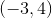?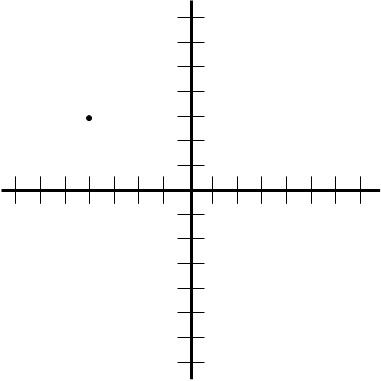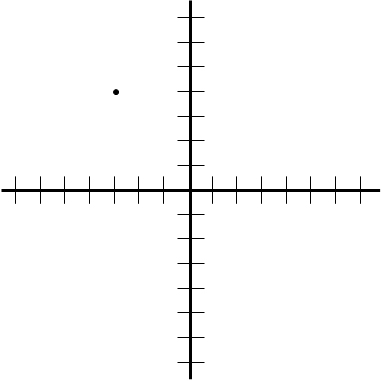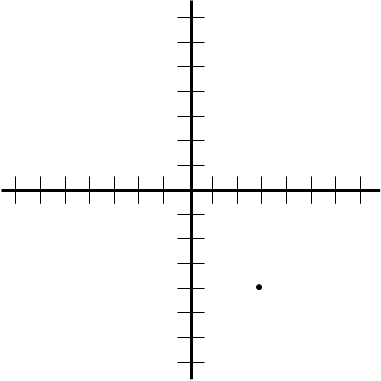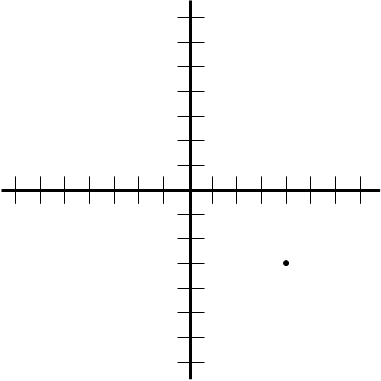Explanation:

The first number,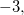indicates how far the point is positioned to the left or right of the origin. Because the number is negative, the point is three units to the LEFT of the origin. The second number indicates how far the point is postioned up or down from the origin. Because the number is positive, the point is located four units above the origin.

### Example Question #2 : How To Graph A Point

The length of line segment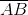is 12 units. If point A is located at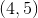, what is a possible location for point B?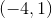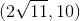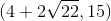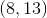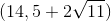Explanation:

To answer this question, we will have to manipulate the distance formula: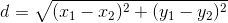To get rid of the square root, we can square both sides: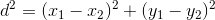and plug in the information given in the question.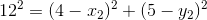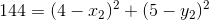At this point we can simply plug in the possible values to determine which combination of coordinates will make the equation above true.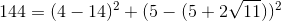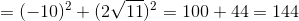Thus the correct coordinate is,.

### Example Question #3 : How To Graph A Point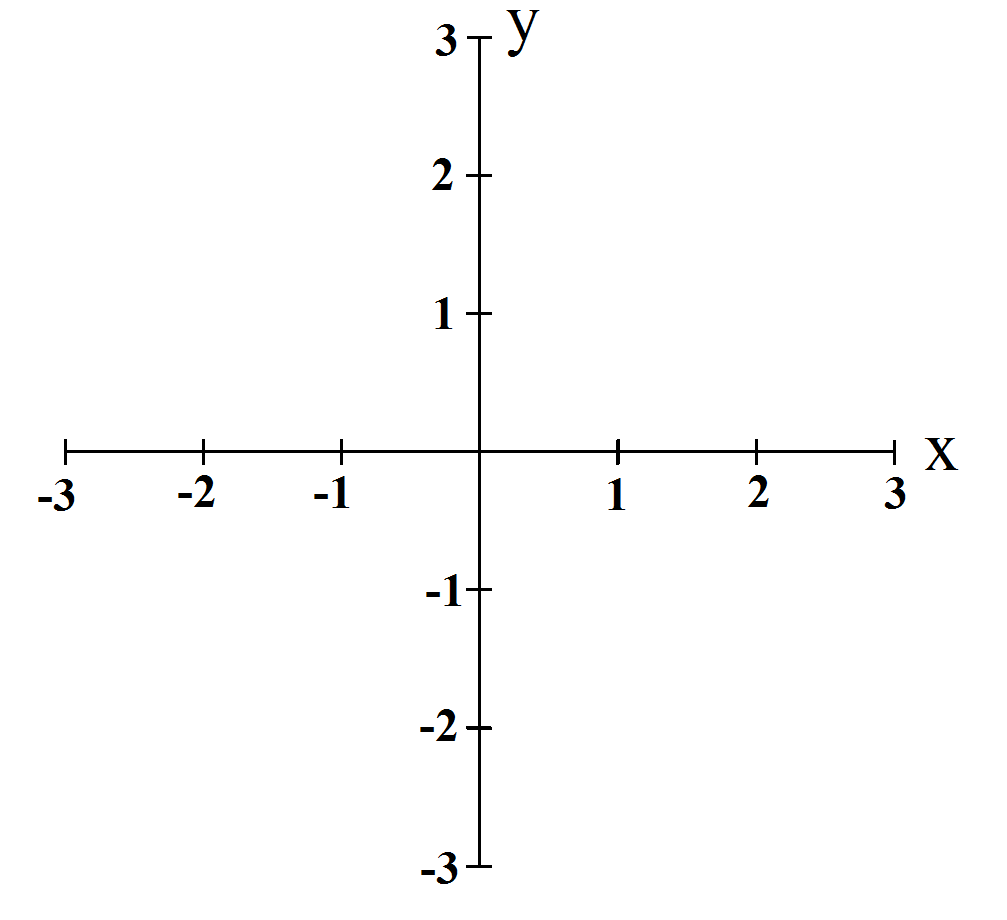Graph the following 4 points. They will be displayed as (x,y) pairs.

(A)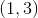(B)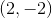(C)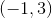(D)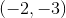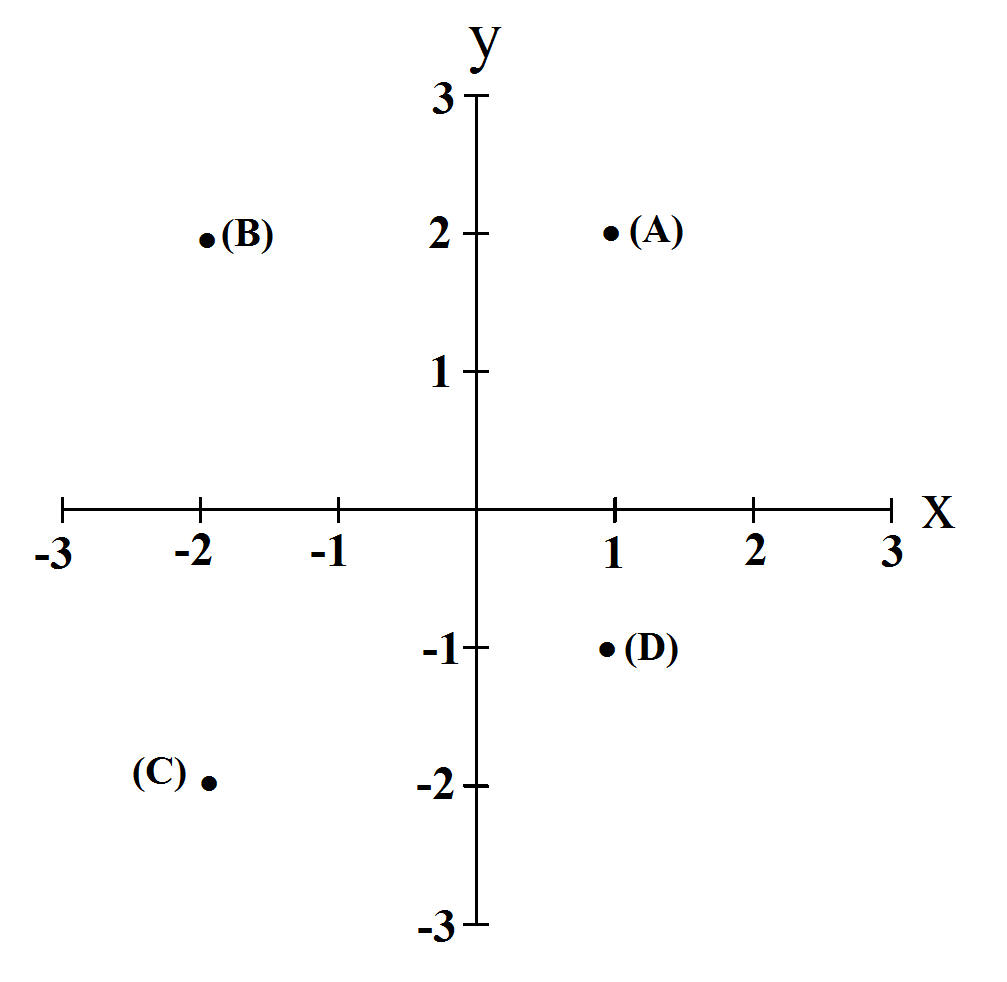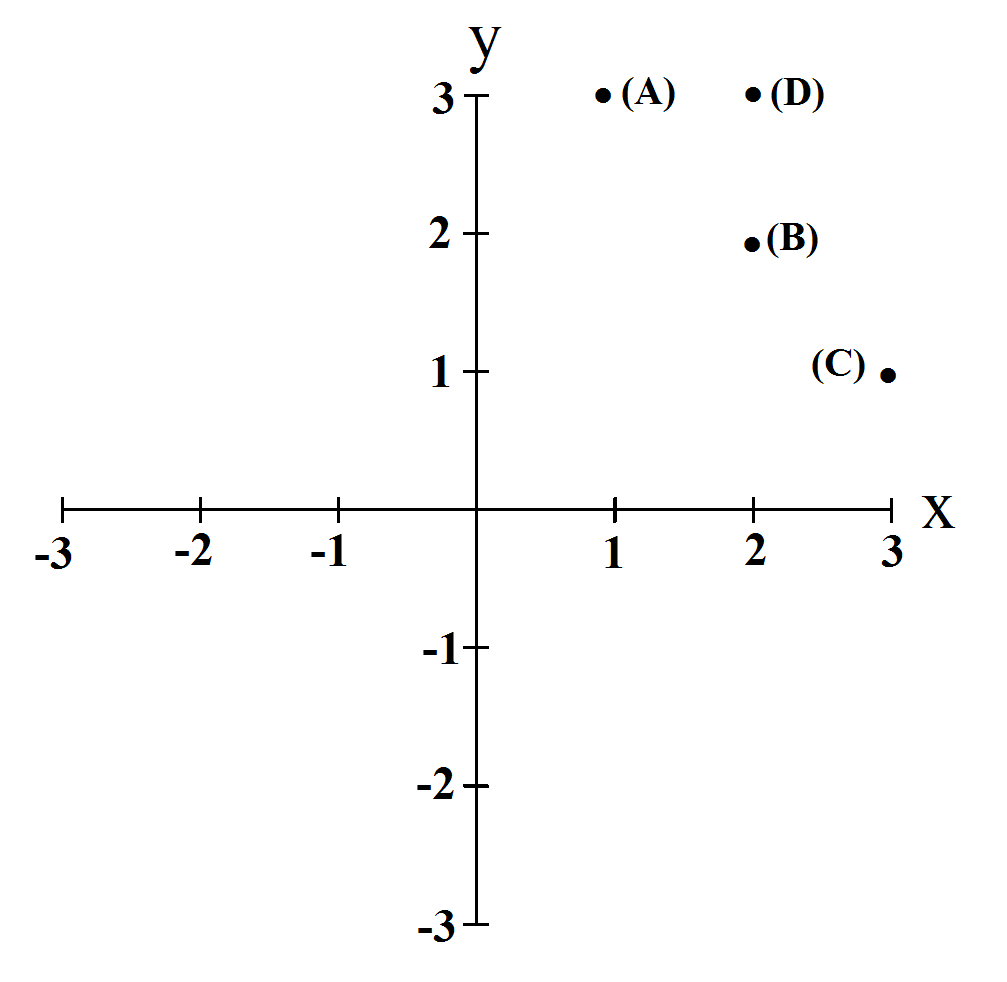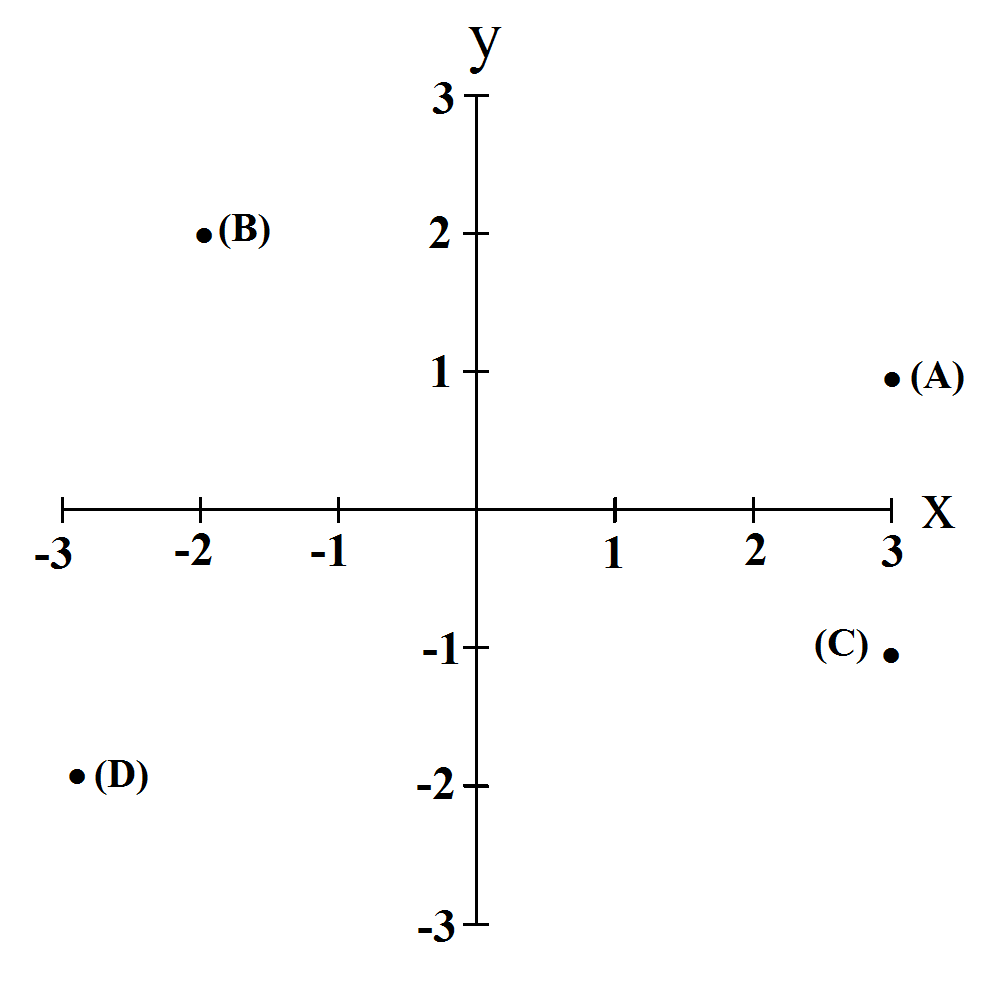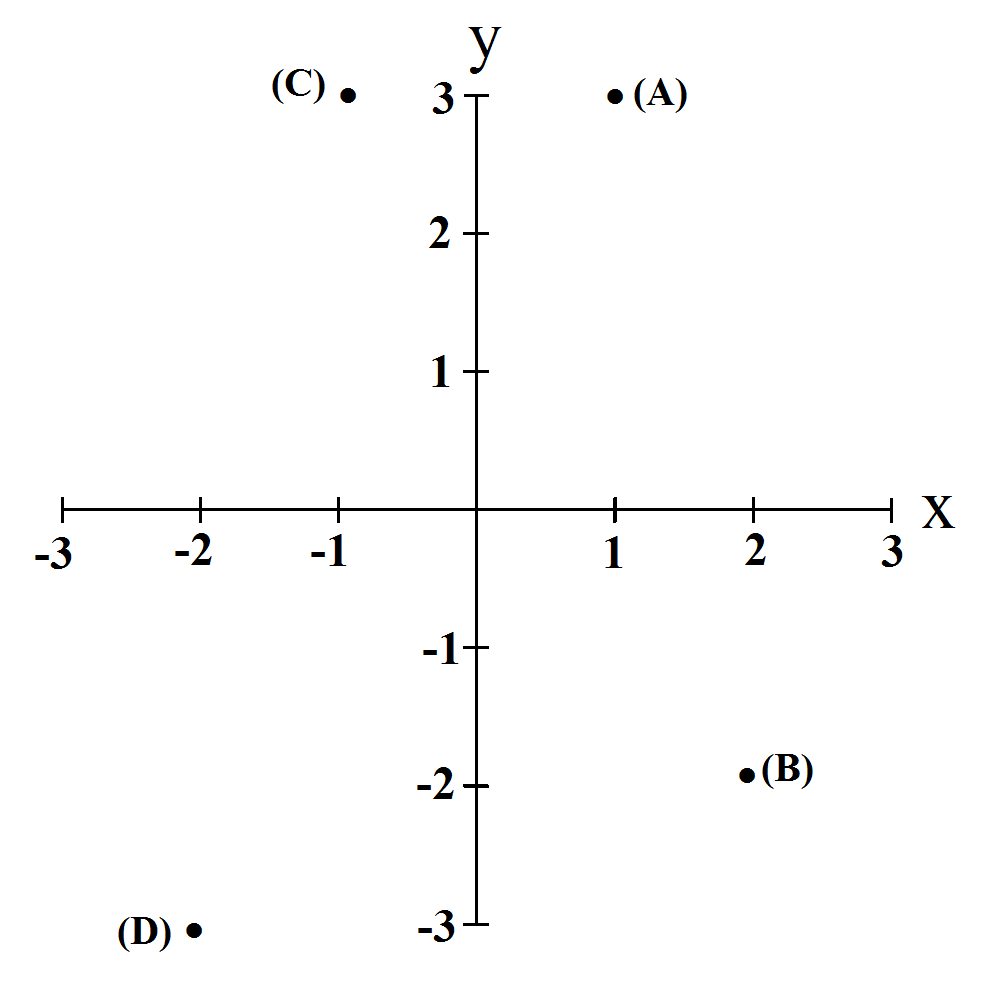Explanation:

To graph these points we just need to remember that the first number is the x value and the second number is the y value. For (A) we have (1,3). So we move one tick over on the positive x-axis. Then from there we move up to the third tick on the y axis: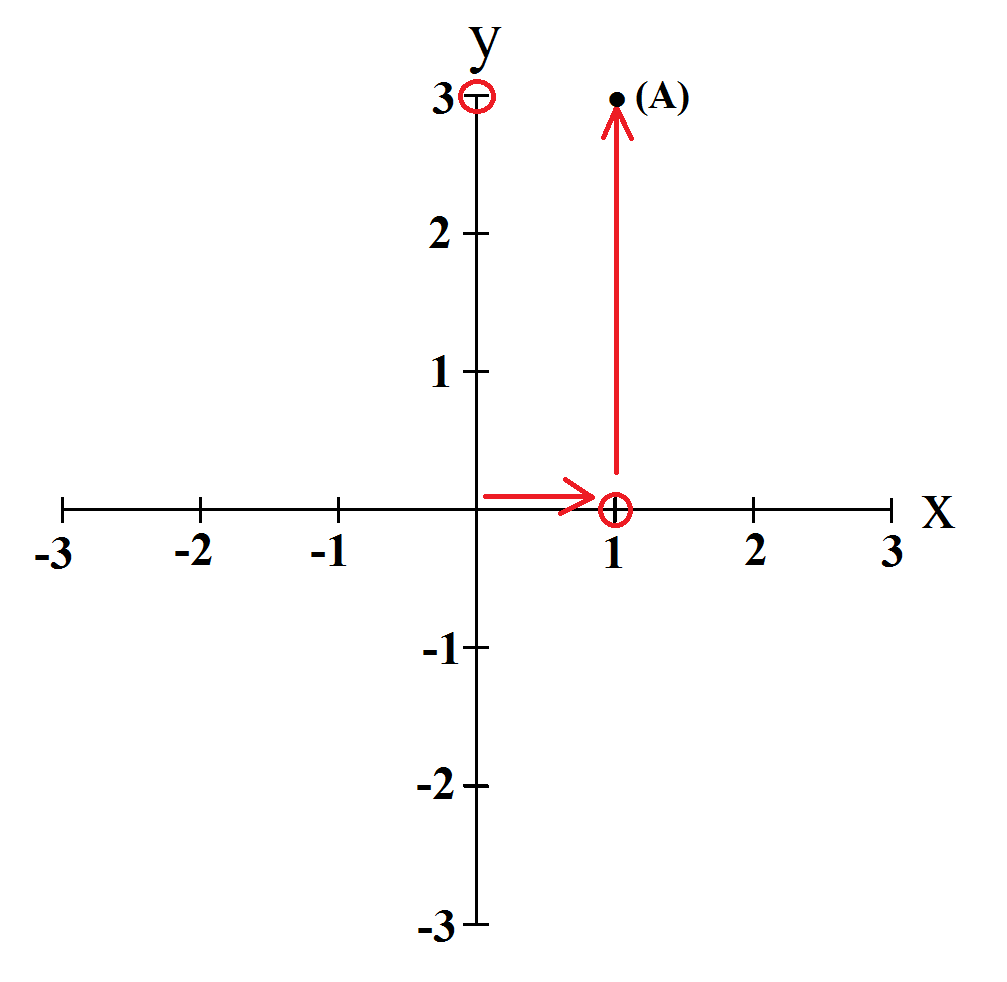If the value is negative we must move in the other direction. So, for all 4 points,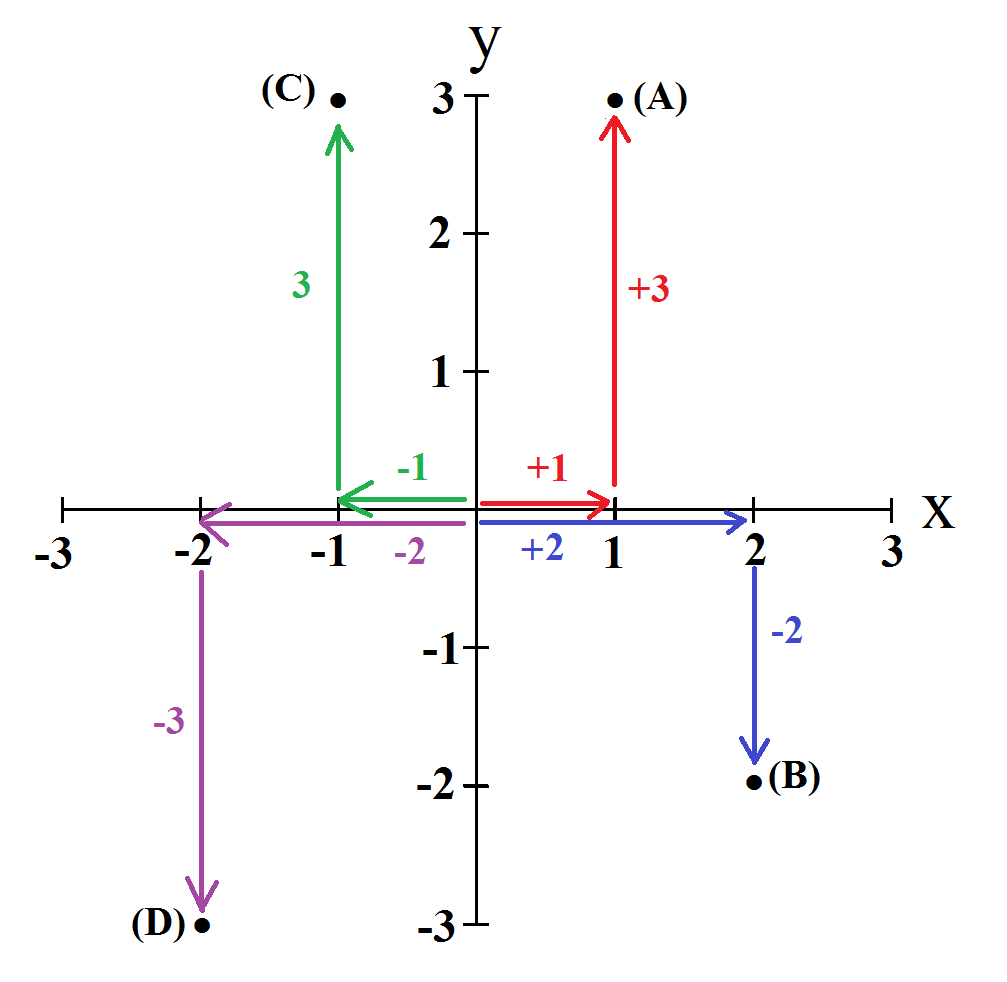### Example Question #1 : How To Graph A Point

What are the coordinates of the point on the given graph?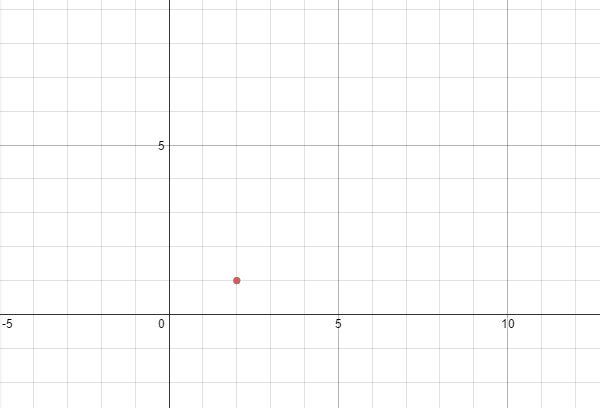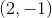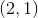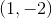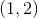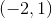Explanation:

When trying to determine coordinates of a point you need to look at the value of x first (how many units left or right the point is, then the y-value (how many up or down it is).

When you look at this point you see that it is moved right 2 units and up 1.